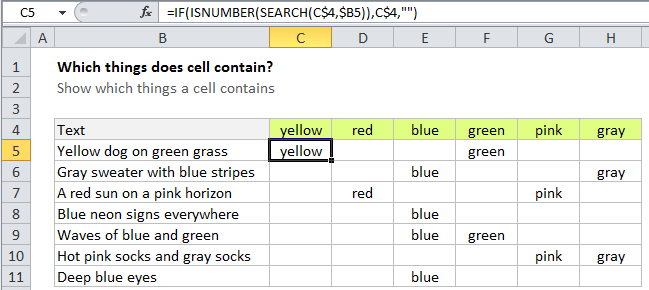## Excel Office

Excel How Tos, Tutorials, Tips & Tricks, Shortcuts

# How to check cell contains which things in Excel

If you have a list of things (words, substrings, etc) and want to find out which of these things appear in a cell, you can build a simple table and use a formula based on the SEARCH function.

### Setup

Suppose you have a cells that contain text that mentions various colors, and you want to figure out which colors are mentioned in each cell.

With the text on the left, create a simple table with the cells you want to check on the left, and the words you want to look for as column labels to the right.

Also See:   If cell is greater than in Excel

See example below:

## Formula

`=IF(ISNUMBER(SEARCH(substring,text)),substring,"")`Note that the references in this formula are carefully constructed. The reference to C\$4 has the row locked, and the reference to \$B5 has the column locked. This allows the formula to be copied throughout the table and still work correctly.

## Explanation

In the example, we are checking for 6 colors, so the table has 7 columns total including the column we are searching within.

Also See:   Excel Data validation require unique number

In the second column, add the first formula. In cell C5 of the example, we have:

`=IF(ISNUMBER(SEARCH(C\$4,\$B5)),C\$4,"")`

### How this formula works

This formula is based on the simpler formula described here. It uses the SEARCH function to look inside B5 for using cell C4 as the search string. If SEARCH returns a number, it means the search string was found. In that case, ISNUMBER will return TRUE, and the IF function will return the value in C4.

Also See:   nth largest value with criteria in Excel

If the search string isn’t found, SEARCH returns an error, ISNUMBER returns FALSE, and the IF function returns an empty string (“”) — the value if FALSE.